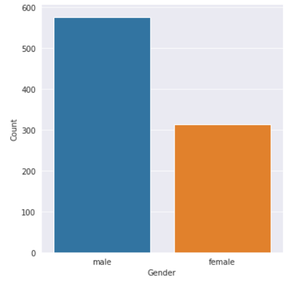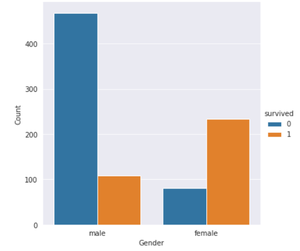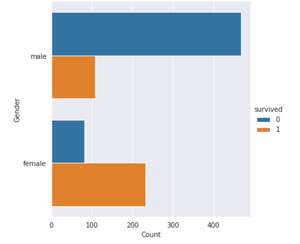# How to Make Countplot or barplot with Seaborn Catplot?

• Last Updated : 25 Nov, 2020

In Python, we can normally plot barcharts for numerical variables. But when it comes to the case of categorical variables then we cannot normally plot the count for each category. Here comes Seaborn Catplot in the picture. It allows you to plot the count of each category for non-numerical/categorical variables.

## Creating the Countplot

Countplot gives a graphical visual for the count of observations in each category using bars. This cannot be used for quantitative variables. It can be created by passing the count value to the kind parameter.

Example: Let’s take an example of a titanic dataset.

## Python3

 `# import libraries ` `import` `matplotlib.pyplot as plt ` `import` `seaborn as sns ` ` `  `# setting background style ` `sns.set_style(``'darkgrid'``) ` ` `  `# import dataset ` `data ``=` `sns.load_dataset(``'titanic'``) ` `data.head() `

Output:Example 1: Creating the countplot

## Python3

 `# plot for count of passengers belonging ` `# to each gender ` `sns.catplot(x``=``'sex'``, kind``=``'count'``, data``=``data) ` `plt.xlabel(``"Gender"``) ` `plt.ylabel(``"Count"``) `

Output:Example 2: Creating the grouped countplot

## Python3

 `# Grouped Countplot/Barplot ` `# Count of passengers who survived ` `# or didn't of each gender ` `sns.catplot(x``=``'sex'``, hue``=``'survived'``, ` `            ``kind``=``'count'``, data``=``data) ` ` `  `plt.xlabel(``"Gender"``) ` `plt.ylabel(``"Count"``) `

Output:Example 3: Creating the horizontal countplot

## Python3

 `# Plotting horizontally ` `sns.catplot(y``=``'sex'``, hue``=``'survived'``,  ` `            ``kind``=``'count'``, data``=``data) ` ` `  `plt.xlabel(``"Count"``) ` `plt.ylabel(``"Gender"``) `

Output:My Personal Notes arrow_drop_up
Recommended Articles
Page :